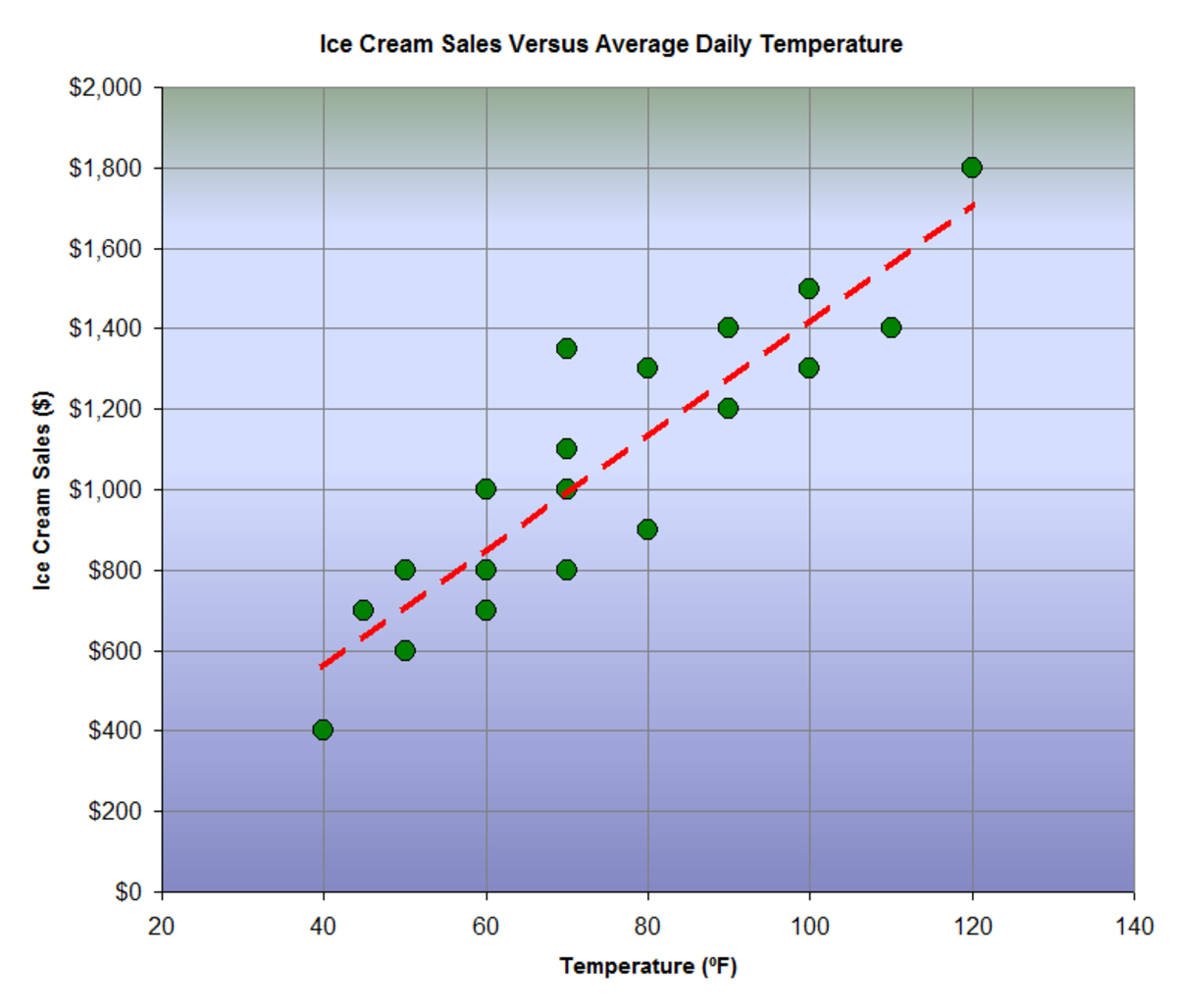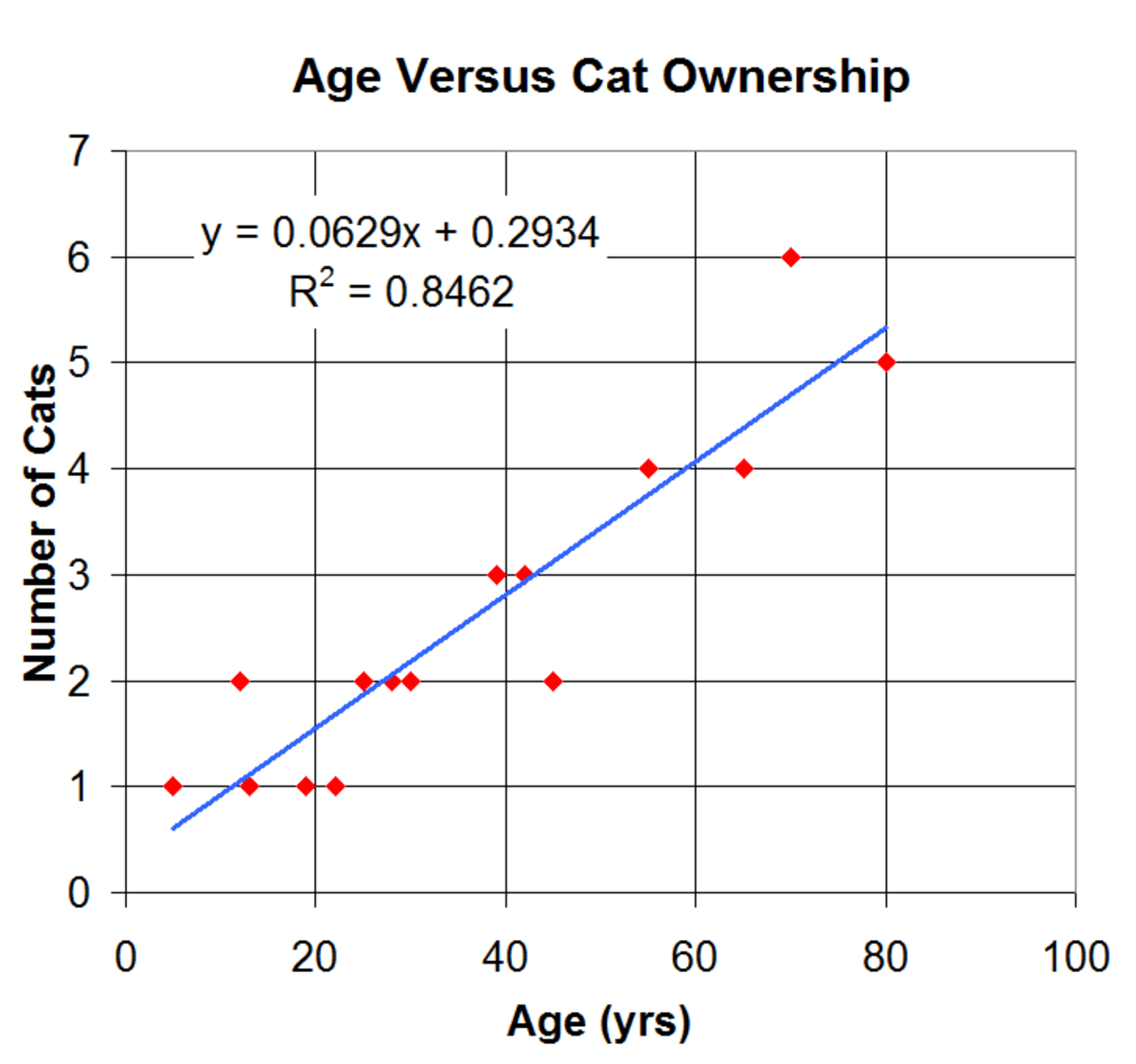# How to Create Your Own Simple Linear Regression Equation

Chris has a Master's degree in engineering and uses his knowledge to write about a variety of topics from an analytical perspective.The relationship between ice cream sales and the outdoor temperature can be represented with a simple regression equation.CWanamaker

Regression equations are frequently used by scientists, engineers, and other professionals to predict a result based on a given input serived from a set of experiment or observation-based data. There are many types of regression equations, but the simplest one is the linear regression equation.

A linear regression equation is simply the equation of a line that is the “best fit” for a particular set of data. Even though you may not be a scientist, engineer, or mathematician, simple linear regression equations can find good uses in anyone’s daily life.

## What Is a Linear Regression Equation?

A linear regression equation takes the same form as the equation of a line, and it's often written in the following general form: y = A + Bx

Here, ‘x’ is the independent variable (your known value), and ‘y’ is the dependent variable (the predicted value). The letters ‘A’ and ‘B’ represent constants that describe the y-axis intercept and the slope of the line.A scatter plot and regression equation of age versus cat ownershipCWanamaker

The image at right shows a set of data points and a “best fit” line that is the result of a regression analysis. As you can see, the line does not actually pass through all of the points. The distance between any point (observed or measured value) and the line (predicted value) is called the error. The smaller the errors are, the more accurate the equation is and the better it is at predicting unknown values. When the errors are reduced to their smallest level possible, the line of ‘best fit' is created.

If you have a spreadsheet program such as Microsoft Excel, then creating a simple linear regression equation is a relatively easy task. After you have input your data into a table format, you can use the chart tool to make a scatter plot of the points. Next, simply right-click on any data point and select “add trend line” to bring up the regression equation dialogue box. Select the linear trend line for the type. Go to the 'options' tab and be sure to check the boxes to display the equation on the chart. Now you can use the equation to predict new values whenever you need to.

Not everything in the world is going to have a linear relationship between them. Many things are better described using exponential or logarithmic equations rather than linear equations. However, that doesn’t preclude any of us from trying to describe something simply. What really matters here is how accurately the linear regression equation describes the relationship between the two variables. If there is a good correlation between the variables, and the relative error is small, then the equation is deemed accurate and can be used to make predictions about new situations.

## What if I don’t have a Spreadsheet or Statistics Program?

Even if you don’t have a spreadsheet program like Microsoft Excel, you can still derive your own regression equation from a small dataset with relative ease (and a calculator). Here is how you do it:

1. Create a table using the data that you have recorded from either observation or an experiment. Label the independent variable ‘x’ and the dependent variable ‘y’

2. Next, add 3 more columns to your table. The first column should be labeled ‘xy’ and should reflect the product of the ‘x’ and ‘y’ values in your first two columns, The next column should be labeled ‘x2’and should reflect the square of the ‘x’ value. The final column should be labeled ‘y2’ and reflect the square of the ‘y’ value.

3. After you have added the three additional columns, you should add a new row to the bottom that totals the values of the numbers in the column above it. When you are done you should have a completed table that looks similar to the one below:

#X (Age)Y (Cats)XYX^2Y^2

1

25

2

50

625

4

2

30

2

60

900

4

3

19

1

19

361

1

4

5

1

5

25

1

5

80

5

400

6400

25

6

70

6

420

4900

36

7

65

4

260

4225

16

8

28

2

56

784

4

9

42

3

126

1764

9

10

39

3

117

1521

9

11

12

2

24

144

4

12

55

4

220

3025

16

13

13

1

13

169

1

14

45

2

90

2025

4

15

22

1

22

484

1

Sum

550

39

1882

27352

135

4. Next, use the following two equations to calculate what the constants ‘A’ and ‘B’ are in the linear equation. Note that from the above table ‘n’ is the sample size (number of data points) which in this case is 15.

Scroll to Continue

## Read More From Owlcation

In the above example relating age to cat ownership, if we use the equations shown above, we get A = 0.29344962 and B = 0.0629059. Therefore our linear regression equation is Y = 0.293 + 0.0629x. This matches the equation that was generated from Microsoft Excel (see the scatter plot above).

As you can see, creating a simple linear regression equation is very easy, even when it is completed by hand.

## How Accurate is my Regression Equation?

When talking about regression equations you may hear about something called the Coefficient of Determination (or R2 value). This is a number between 0 and 1 (basically a percentage) that tells you how well the equation actually describes the set of data. The closer the R2 value is to 1, the more accurate the equation is. Microsoft Excel can calculate the R2 value for you very easily. There is a way to calculate the R2 value by hand but it is quite tedious. Perhaps that will be another article that I will write in the future.

## Examples of Other Potential Applications

In addition to the above example, there are several other things that regression equations can be used for. In fact, the list of possibilities is endless. All that is really needed is a desire to represent the relationship of any two variables with a linear equation. Below is a brief list of possible applications of regression equations.

• Comparing the amount of money spent on Christmas gifts given the number of people you have to buy for.
• Comparing the amount of food needed for dinner given the number of people that are going to eating
• Describing the relationship between how much TV you watch and how many calories you consume
• Describing how the amount times you do laundry relates to the length of time clothes remain wearable
• Describing the relationship between the average daily temperature and the amount of people seen at the beach or a park
• Describing how your electricity usage relates to the average daily temperature
• Correlating the amount of birds observed in your backyard with the amount of birdseed that you left outside
• Relating the size of a house with the amount of electricity that is needed to operate and maintain it
• Relating the size of a house with the price for a given location
• Relating the height versus the weight of everyone in your family

These are just a few of the endless things that regression equations can be used for. As you can see, there are many practical applications for these equations in our everyday life. Wouldn’t it be great to make reasonably accurate predictions about various things that we experience each and every day? I sure think so! Using this relatively simple mathematical procedure, I hope that you’ll find new ways to bring order to things that would otherwise be described as unpredictable.

## Questions & Answers

Question: Q1. The following table represent a set of data on two variables Y and X. (a) Determine the linear regression equation Y = a + bX. Use your line to estimate Y when X = 15. (b) Calculate the Pearson’s correlation coefficient between the two variables. (c) Calculate Spearman's correlation Y 5 15 12 6 30 6 10 X 10 5 8 20 2 24 8?

Answer: Given the set of numbers Y = 5,15,12,6,30,6,10 and X = 10,5,8,20,2,24,8 the equation of a simple linear regression model becomes: Y = -0.77461X +20.52073.

When X is equal to 15, the equation predicts a Y value of 8.90158.

Next, to calculate the Pearson Correlation Coefficient, we use the equation r = (sum(x-xbar)(y-ybar))/(root(sum(x-xbar)^2 sum(y-ybar)^2)).

Next, inserting values, the equation becomes r = (-299)/(root((386)(458))) = -299/420.4617,

Therefore, Pearson's Correlation Coefficient is -0.71112

Finally, to calculate Spearman's Correlation, we use the following equation: p = 1 - [((6(sum(d^2))/(n(n^2-1))]

To use the equation we first rank the data, calculate the difference in rank as well as the squared difference in rank. The sample size, n, is 7 and the sum of the square of rank differences is 94

Solving p = 1 - ((6)(94))/(7(7^2-1) = 1 - (564)/(336) = 1 - 1.678571 = -0.67857

Therefore, Spearman's Correlation is -0.67857

abdullah imtiaz on April 11, 2020:

why calculate y2 when we don't even use it

Bob longnecker on February 16, 2020:

If I have a right triangle with the following given:

A = 3.6

Three angles are 90, 1 and 89.

What is the length of side B ?